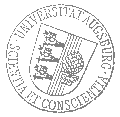# Universität AugsburgInstitut für MathematikAnalysis-Seminar Augsburg-München

Professor Dr. Caterina Zeppieri
Universität Münster

spricht am

Donnerstag, 15. Juni 2023

um

15:00 Uhr

im

Raum 2004 (L1)

über das Thema:

## »Homogenisation of nonlinear randomly perforated media«

 Abstract: In this talk we discuss the homogenisation of integral functionals with $q$ growth in a bounded domain of $\mathbb R^n$, $n>q>1$, which is perforated by a random number of small spherical holes with random radii and centers. We show that for a class of stationary short-range correlated measures for the centres and radii of the holes, in the homogenised limit we obtained a nonlinear averaged analogue of the strange term'' obtained by Cioranescu and Murat in 1982 in the periodic case. In our case we only require that the random radii have finite $(n-q)$-moment, which is the minimal assumption to ensure that the expectation of the nonlinear capacity of the balls is finite. Although under this assumption there are holes which overlap with probability one, we can prove that the clustering holes do not have any impact on the homogenisation procedure and the limit functional. This is a joint work with K. Zemas (University of Muenster) and L. Scardia (Heriot Watt University).

 Hierzu ergeht herzliche Einladung. Prof. Dr. Lisa Beck

[Impressum]      [Datenschutz]      wwwadm@math.uni-augsburg.de,     Mi 7-Jun-2023 09:22:23 MESZ